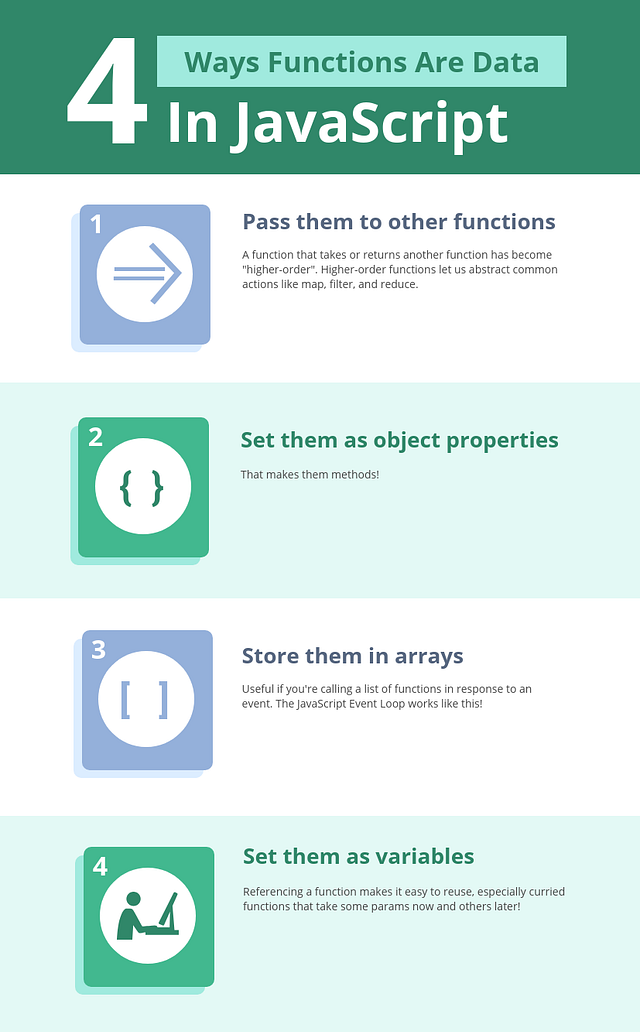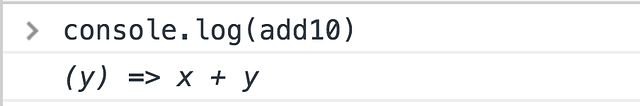# An introduction to Higher-Ordaer Functions in JavaScriptIn this tutorial we will learn what higher order functions are, how and why is it possible to use them in Javascript.

In this tutorial we will learn what higher order functions are, how and why is it possible to use them in Javascript.

Use functions as data, and unlock some powerful patterns.

### Higher-Order Functions

A function that accepts and/or returns another function is called a higher-order function.

It’s higher-order because instead of strings, numbers, or booleans, it goes higher to operate on functions. Pretty meta.

With functions in JavaScript, you can

• Store them as variables
• Use them in arrays
• Assign them as object properties (methods)
• Pass them as arguments
• Return them from other functions

Like any other piece of data. That’s the key here.

### Functions Operate on Data

#### Strings Are Data

``````sayHi = (name) => `Hi, \${name}!`;
result = sayHi('User');

console.log(result); // 'Hi, User!'
``````

#### Numbers Are Data

``````double = (x) => x * 2;
result = double(4);

console.log(result); // 8
``````

#### Booleans Are Data

``````getClearance = (allowed) => allowed ?
'Access granted' :

result1 = getClearance(true);
result2 = getClearance(false);

console.log(result1); // 'Access granted'
``````

#### Objects Are Data

``````getFirstName = (obj) => obj.firstName;
result = getFirstName({
firstName: 'Yazeed'
});

console.log(result); // 'Yazeed'
``````

#### Arrays Are Data

``````len = (array) => array.length;
result = len([1, 2, 3]);

console.log(result); // 3
``````

These 5 types are first-class citizens in every mainstream language.

What makes them first-class? You can pass them around, store them in variables and arrays, use them as inputs for calculations. You can use them like any piece of data.

### Functions Can Be Data Too#### Functions as Arguments

``````isEven = (num) => num % 2 === 0;
result = [1, 2, 3, 4].filter(isEven);

console.log(result); // [2, 4]
``````

See how `filter` uses `isEven` to decide what numbers to keep? `isEven`, a function, was a parameter to another function.

It’s called by `filter` for each number, and uses the returned value `true` or `false` to determine if a number should be kept or discarded.

#### Returning Functions

``````add = (x) => (y) => x + y;
``````

`add` requires two parameters, but not all at once. It’s a function asking for just `x`, that returns a function asking for just `y`.

Again, this is only possible because JavaScript allows functions to be a return value — just like strings, numbers, booleans, etc.

You can still supply `x` and `y` immediately, if you wish, with a double invocation

``````result = add(10)(20);

console.log(result); // 30
``````

Or `x` now and `y` later:

``````add10 = add(10);

console.log(result); // 30
``````

Let’s rewind that last example. `add10` is the result of calling `add` with one parameter. Try logging it in the console.`add10` is a function that takes a `y` and returns `x + y`. After you supply `y`, it hurries to calculate and return your end result.### Greater Reusability

Probably the greatest benefit of HOFs is greater reusability. Without them, JavaScript’s premiere Array methods — `map`, `filter`, and `reduce` — wouldn’t exist!

Here’s a list of users. We’re going to do some calculations with their information.

``````users = [{
name: 'Yazeed',
age: 25
}, {
name: 'Sam',
age: 30
}, {
name: 'Bill',
age: 20
}];
``````

#### Map

Without higher-order functions, we’d always need loops to mimic `map`'s functionality.

``````getName = (user) => user.name;

for (let i = 0; i < users.length; i++) {
const name = getName(users[i]);

}

// ["Yazeed", "Sam", "Bill"]
``````

Or we could do this!

``````usernames = users.map(getName);

// ["Yazeed", "Sam", "Bill"]
``````

#### Filter

In a HOF-less world, we’d still need loops to recreate `filter`'s functionality too.

``````startsWithB = (string) => string
.toLowerCase()
.startsWith('b');

namesStartingWithB = [];

for (let i = 0; i < users.length; i++) {
if (startsWithB(users[i].name)) {
namesStartingWithB.push(users[i]);
}
}

console.log(namesStartingWithB);
// [{ "name": "Bill", "age": 20 }]
``````

Or we could do this!

``````namesStartingWithB = users
.filter((user) => startsWithB(user.name));

console.log(namesStartingWithB);
// [{ "name": "Bill", "age": 20 }]
``````

#### Reduce

Yup, reduce too… Can’t do much cool stuff without higher-order functions!! 😁

``````total = 0;

for (let i = 0; i < users.length; i++) {
total += users[i].age;
}

console.log(total);
// 75
``````

How’s this?

``````totalAge = users
.reduce((total, user) => user.age + total, 0);

console.log(totalAge);
// 75
``````

### Summary

• Store them as variables
• Use them in arrays
• Assign them as object properties (methods)
• Pass them as arguments
• Return them from other functions

## The essential JavaScript concepts that you should understand

The essential JavaScript concepts that you should understand - For successful developing and to pass a work interview

## Data Types In JavaScript

JavaScript data types are kept easy. While JavaScript data types are mostly similar to other programming languages; some of its data types can be unique. Here, we’ll outline the data types of JavaScript.

## JavaScript Memory Management System

The main goal of this article is help to readers to understand that how memory management system performs in JavaScript. I will use a shorthand such as GC which means Garbage Collection. When the browsers use Javascript, they need any memory location to store objects, functions, and all other things. Let’s deep in dive that how things going to work in GC.

## Create a Line Through Effect with JavaScript

In this post we are going to create an amazing line through effect, with help of CSS and lots of JavaScript. So, head over to your terminal and create a folder LineThroughEffect. Create three files -index.html, main.js and styles.css inside it.

## Grokking Call(), Apply() and Bind() Methods in JavaScript

In this article, we will have a look at the call(), apply() and bind() methods of JavaScript. Basically these 3 methods are used to control the invocation of the function.